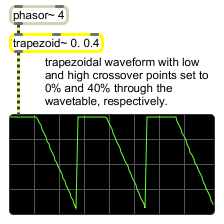# trapezoid~

Trapezoidal wavetable

## Description

trapezoid~ is a trapezoidal wavetable with signal inputs to change ramp up/down phase position. The default lo/hi points are 0. and 1., but may be changed using the lo/hi messages.

## Arguments

Name Type Opt Description
ramp-up and ramp-down float opt Two floating-point values can be used to specify the ramp up and ramp down values. The arguments 0. 0. produce a ramp waveform, and 0.5 0.5 produces a triangle waveform.

## Messages

 float trapezoidal-control [float] In left inlet: Any float or signal or an input signal progressing from 0 to 1 is used to scan the trapezoid~ object's wavetable. The output of a phasor~ or some other audio signal can be used to control trapezoid~ as an oscillator, treating the contents of the wavetable as a repeating waveform. In middle inlet: The ramp up portion of the trapezoidal waveform, specified as a fraction of a cycle between 0 and 1.0. The default is .1. In right inlet: The ramp up portion of the trapezoidal waveform, specified as a fraction of a cycle between 0 and 1.0. The default is 0.9. hi maximum-value [float] In left inlet: The word hi, followed by an optional number, sets the maximum value of trapezoid~ for signal output. The default value is 1.0. lo minimum-value [float] In left inlet: The word lo, followed by an optional number, sets the minimum value of trapezoid~ for signal output. The default value is 0. signal In left inlet: Any float or signal or an input signal progressing from 0 to 1 is used to scan the trapezoid~ object's wavetable. The output of a phasor~ or some other audio signal can be used to control trapezoid~ as an oscillator, treating the contents of the wavetable as a repeating waveform. In middle inlet: The ramp up portion of the trapezoidal waveform, specified as a fraction of a cycle between 0 and 1.0. The default is .1. In right inlet: The ramp up portion of the trapezoidal waveform, specified as a fraction of a cycle between 0 and 1.0. The default is 0.9.

## Output

signal: A signal which corresponds to the value referenced by the trapezoid~ object's input signal. If the output of a phasor~ or some other audio signal is used to scan the trapezoid~ object, the output will be a periodic waveform.

## Examplestrapezoid~ generates a trapezoidal waveform that lets you specify the phase points at which it changes direction